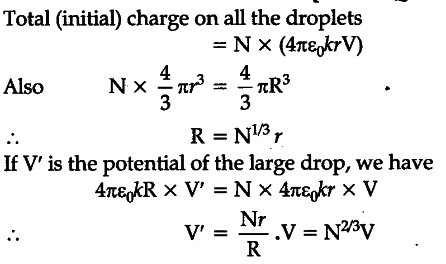# A Non spherical droplets, each of radius r, have been charged to have a potential V each

A Non spherical droplets, each of radius r, have been charged to have a potential V each. If all these droplets were to coalesce to form a single large drop, what would be the potential of this large drop ?
(It is given that the capacitance of a sphere of radius x